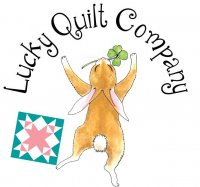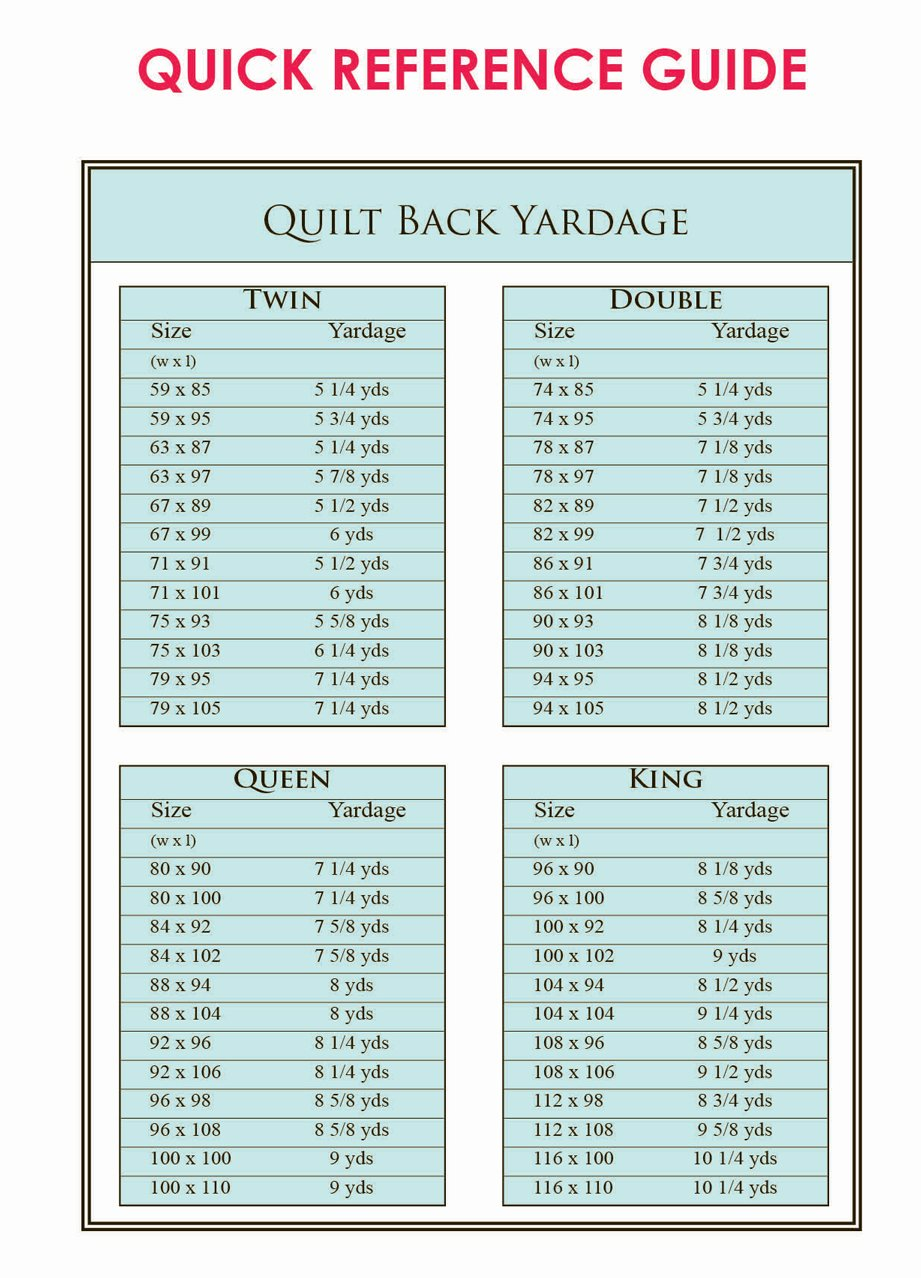Formula to determine the amount of binding fabric needed:

Length x 2
+     Width x 2
=     Total / (divided by) 42 (width of fabric) = # of strips needed (round up to the nearest whole number)

# of strips x 2.5 (width of strips) = total number of inches of fabric needed / (divide by) 36 = yardage needed for binding.

For quilts up to 80" wide, this formula will determine the amount of backing fabric needed. Otherwise, see chart below.

_____ "        (quilt top length)
+              4"       (for extra backing for quilting process)
/             36" (divide by how many inches in a yard)
=     _____  yards
x            2
=    ______   yards backing fabric needed (round up to nearest 1/4 yard.